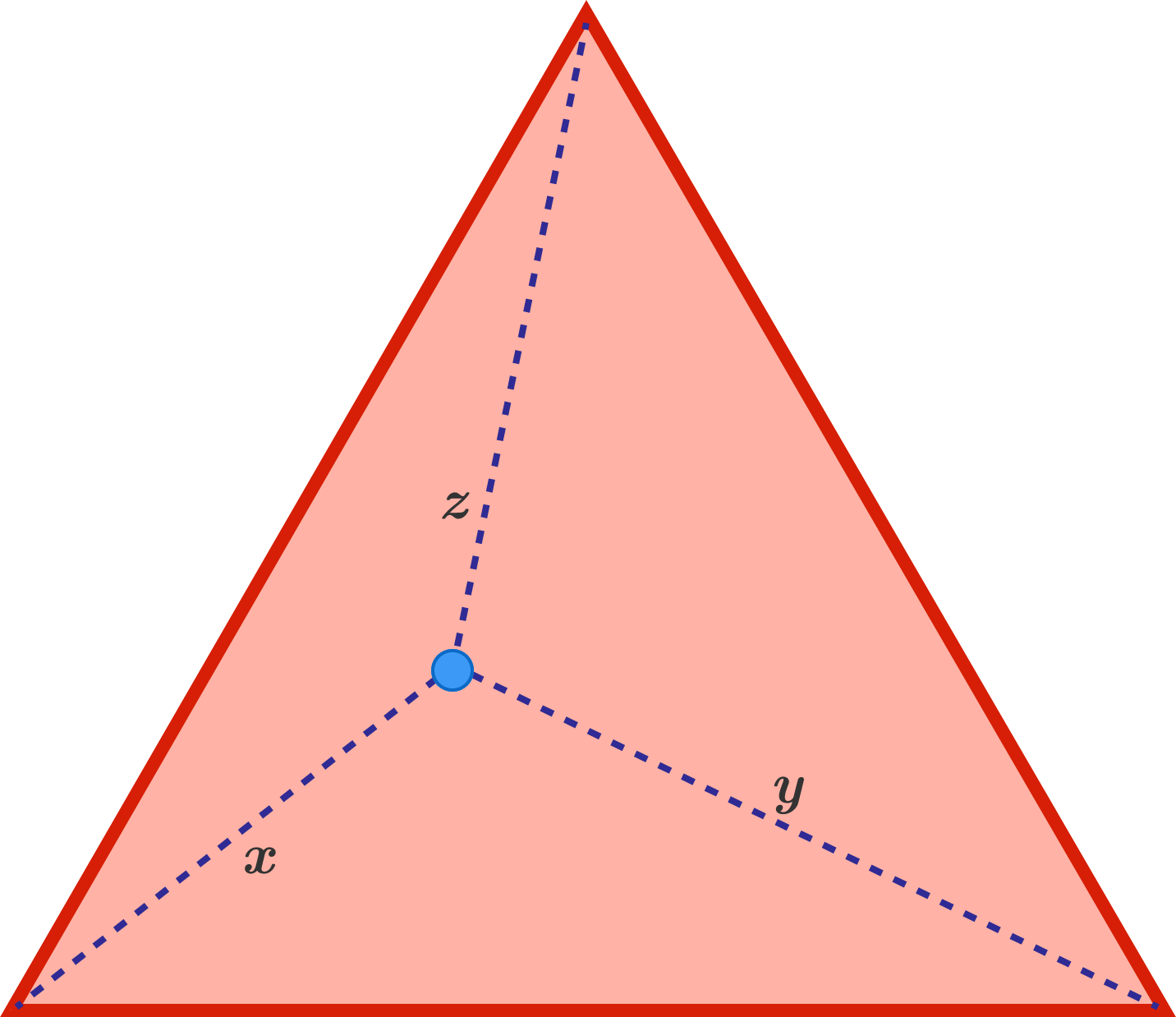# Equilateral

Geometry Level 2

As shown in the diagram, a blue point is randomly chosen inside an equilateral triangle. The distances between the blue point and each of the vertices are $x, y, z$.

Is the following statement true or false?

There exists a triangle with side lengths $x, y, z$.×